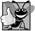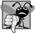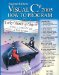# Increment and Decrement Operators

C# provides two unary operators for adding 1 to or subtracting 1 from the value of a numeric variable. These are the unary increment operator, ++, and the unary decrement operator, --, respectively, which are summarized in Fig. 5.15. An application can increment by 1 the value of a variable called c using the increment operator, ++, rather than the expression c = c + 1 or c += 1. An increment or decrement operator that is prefixed to (placed before) a variable is referred to as the prefix increment operator or prefix decrement operator, respectively. An increment or decrement operator that is postfixed to (placed after) a variable is referred to as the postfix increment operator or postfix decrement operator, respectively.

Figure 5.15. Increment and decrement operators.

Operator

Called

Sample expression

Explanation

++

prefix increment

++a

Increment a by 1, then use the new value of a in the expression in which a resides.

++

postfix increment

a++

Use the current value of a in the expression in which a resides, then increment a by 1.

--

prefix decrement

--b

Decrement b by 1, then use the new value of b in the expression in which b resides.

--

postfix decrement

b--

Use the current value of b in the expression in which b resides, then decrement b by 1.

Incrementing (or decrementing) a variable with the prefix increment (or prefix decrement) operator causes it to be incremented (or decremented) by 1, and then the new value of the variable is used in the expression in which it appears. Incrementing (or decrementing) the variable with the postfix increment (or postfix decrement) operator causes the current value of the variable to be used in the expression in which it appears, and then the variable's value is incremented (or decremented) by 1.## Good Programming Practice 5 8

Unlike binary operators, the unary increment and decrement operators should (by convention) be placed next to their operands, with no intervening spaces.

Figure 5.16 demonstrates the difference between the prefix increment and postfix increment versions of the ++ increment operator. The decrement operator (--) works similarly. Note that this example contains only one class, with method Main performing all the class's work. In this chapter and in Chapter 4, you have seen examples consisting of two classesone class containing methods that perform useful tasks and one containing method Main, which creates an object of the other class and calls its methods. In this example, we simply want to show the mechanics of the ++ operator, so we use only one class declaration containing method Main. Occasionally, when it makes no sense to try to create a reusable class to demonstrate a simple concept, we will use a mechanical example contained entirely within the Main method of a single class.

Figure 5.16. Prefix increment and postfix increment operators.

``` 1 // Fig. 5.16: Increment.cs
2 // Prefix increment and postfix increment operators.
3 using System;
4
5 public class Increment
6 {
7 public static void Main( string[] args )
8 {
9 int c;
10
11 // demonstrate postfix increment operator
12 c = 5; // assign 5 to c
13 Console.WriteLine( c ); // print 5
14 Console.WriteLine( c++ ); // print 5 again, then increment
15 Console.WriteLine( c ); // print 6
16
17 Console.WriteLine(); // skip a line
18
19 // demonstrate prefix increment operator
20 c = 5; // assign 5 to c
21 Console.WriteLine( c ); // print 5
22 Console.WriteLine( ++c ); // increment then print 6
23 Console.WriteLine( c ); // print 6 again
24 } // end Main
25 } // end class Increment
```

 ```5 5 6 5 6 6 ```

Line 12 initializes the variable c to 5, and line 13 outputs c's initial value. Line 14 outputs the value of the expression c++. This expression performs the postfix increment operation on the variable c, so c's original value (5) is output, then c's value is incremented. Thus, line 14 outputs c's initial value (5) again. Line 15 outputs c's new value (6) to prove that the variable's value was indeed incremented in line 14.

Line 20 resets c's value to 5, and line 21 outputs c's value. Line 22 outputs the value of the expression ++c. This expression performs the prefix increment operation on c, so its value is incremented, then the new value (6) is output. Line 23 outputs c's value again to show that the value of c is still 6 after line 22 executes.

The arithmetic compound assignment operators and the increment and decrement operators can be used to simplify statements. For example, the three assignment statements in Fig. 5.12 (lines 24, 26 and 29)

```passes = passes + 1;
failures = failures + 1;
studentCounter = studentCounter + 1;
```

can be written more concisely with compound assignment operators as

```passes += 1;
failures += 1;
studentCounter += 1;
```

and even more concisely with prefix increment operators as

```++passes;
++failures;
++studentCounter;
```

or with postfix increment operators as

```passes++;
failures++;
studentCounter++;
```

When incrementing or decrementing a variable in a statement by itself, the prefix increment and postfix increment forms have the same effect, and the prefix decrement and postfix decrement forms have the same effect. It is only when a variable appears in the context of a larger expression that the prefix increment and postfix increment have different effects (and similarly for the prefix decrement and postfix decrement).## Common Programming Error 5 8

Attempting to use the increment or decrement operator on an expression other than one to which a value can be assigned is a syntax error. For example, writing ++(x + 1) is a syntax error because (x + 1) is not a variable.

Figure 5.17 shows the precedence and associativity of the operators we have introduced to this point. The operators are shown from top to bottom in decreasing order of precedence. The second column describes the associativity of the operators at each level of precedence. The conditional operator (?:); the unary operators prefix increment (++), prefix decrement (--), plus (+) and minus (-); the cast operators; and the assignment operators =, +=, -=, *=, /= and %= associate from right to left. All the other operators in the operator precedence chart in Fig. 5.17 associate from left to right. The third column names the groups of operators.

Figure 5.17. Precedence and associativity of the operators discussed so far.

(This item is displayed on page 205 in the print version)

Operators

Associativity

Type

```. new ++(postfix) --(postfix)
```

left to right

highest precedence

```++ -- + - (type)
```

right to left

unary prefix

```* / %
```

left to right

multiplicative

```+ -
```

left to right

```< <= > >=
```

left to right

relational

```== !=
```

left to right

equality

```?:
```

right to left

conditional

```= += -= *= /= %=
```

right to left

assignmentVisual C# 2005 How to Program (2nd Edition)
ISBN: 0131525239
EAN: 2147483647
Year: 2004
Pages: 600

Similar book on Amazon